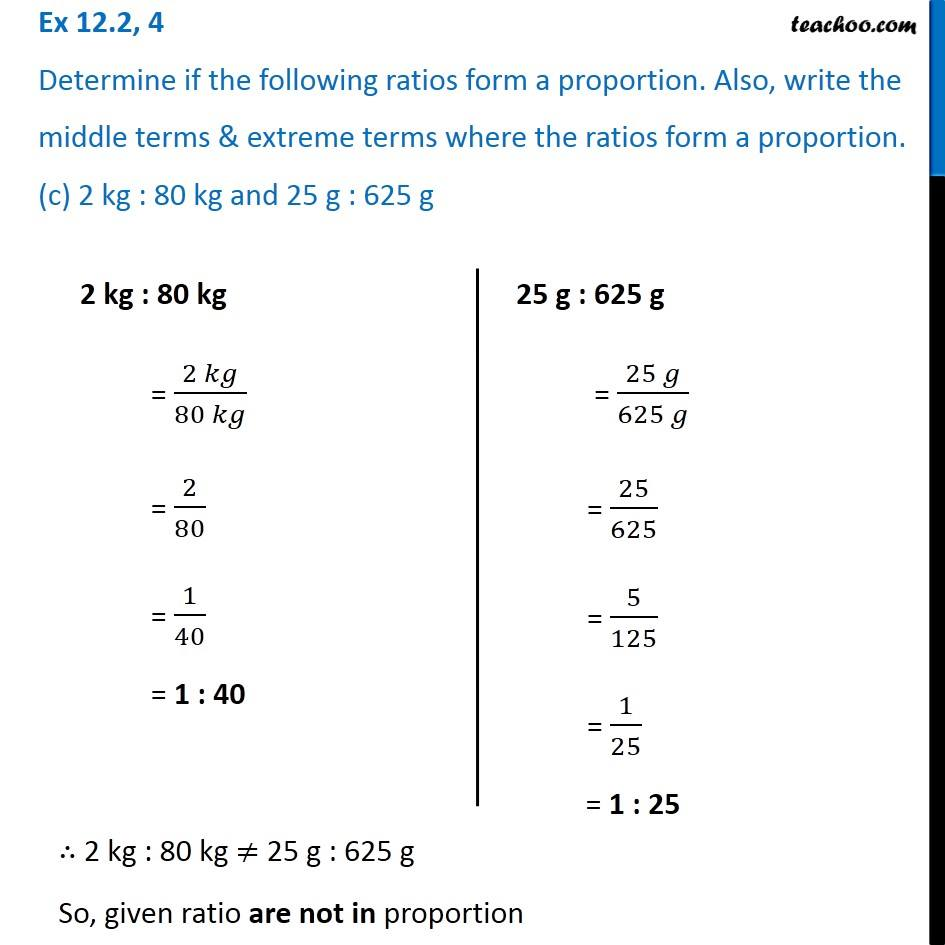Ex 12.2

Chapter 12 Class 6 Ratio and Proportion
Serial order wiseLearn in your speed, with individual attention - Teachoo Maths 1-on-1 Class

### Transcript

Ex 12.2, 4 Determine if the following ratios form a proportion. Also, write the middle terms & extreme terms where the ratios form a proportion. (c) 2 kg : 80 kg and 25 g : 625 g2 kg : 80 kg = (2 𝑘𝑔)/(80 𝑘𝑔) = 2/80 = 1/40 = 1 : 40 25 g : 625 g = (25 𝑔)/(625 𝑔) = 25/625 = 5/125 = 1/25 = 1 : 25 ∴ 2 kg : 80 kg ≠ 25 g : 625 g So, given ratio are not in proportion